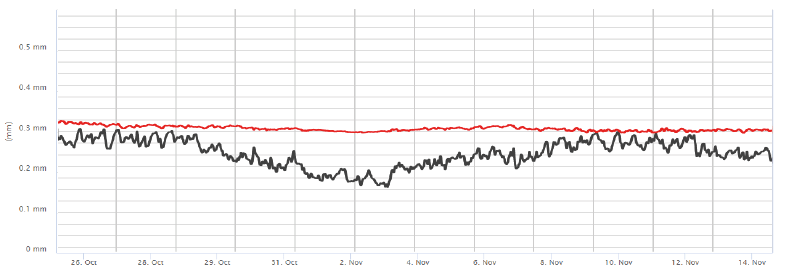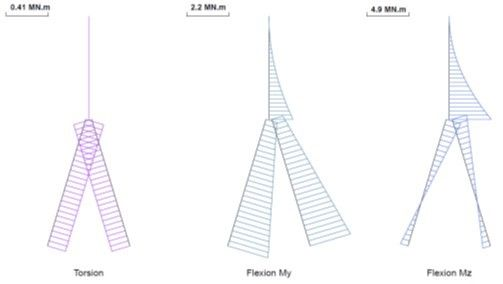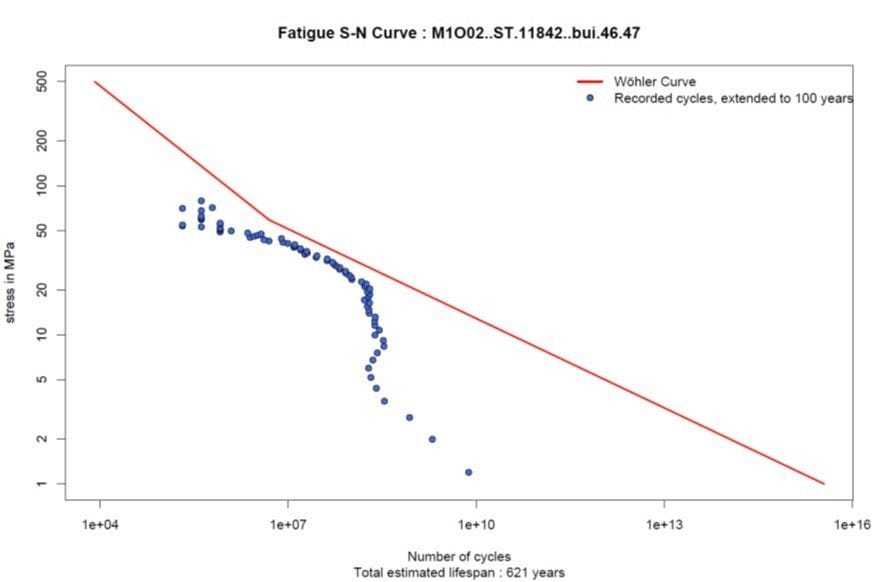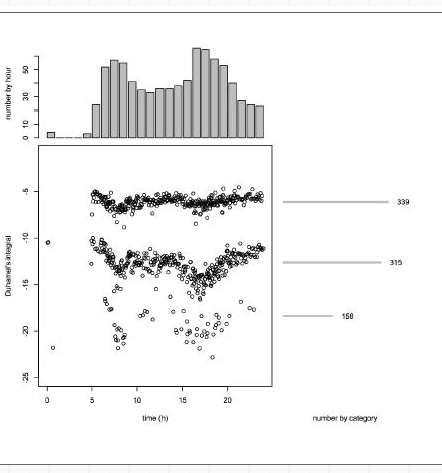We develop and produce our own analytical tools and then test and validate new data processing methods, which allows us to constantly innovate, in terms of both our processes and the quality of information we provide.

##### Basic analysis

A basic analysis, showing average values or event counts: deformation of materials, the structure’s angle of inclination, temperatures, etc.

Algorithms used to distinguish between the different effects impacting the structure and to detect anomalies, in order to derive a better knowledge of them, using measurement-based correlation models.

##### Predictive analysis

Extensive knowledge obtained through large-scale statistical analysis: advanced inverse modeling, leading to accurate lifetime assessments, maintenance optimization and cost deferrals.

## THERMAL CORRECTION

The algorithmic element of thermal correction permits to automatically subtract the (static) deformation measurements of the OSMOS Optical Strands from the effect of the surrounding temperature. In its current version, the algorithmic element has an automatic management of the learning period of the strain-temperature relationship, the calculation of the thermal correction, an estimation of the confidence intervals of this correction and the restitution. a note from the sensor.

###### SPECIFIC LONG-TERM EVOLUTION## WEIGH-IN-MOTION

The weighing and counting algorithm uses an analysis of the dynamic measurements taken by the OSMOS Optical Strands to count the convoys passing over a bridge. The algorithm also evaluates the weights of those convoys, based on the dynamic measurements and on calibration data from the test phase (supervised by the engineers).

###### WEIGH-IN-MOTION## DESCRIPTIVE QUANTITIES

The algorithm used to calculate descriptive quantities automatically processes the dynamic deformation measurements taken by the OSMOS Optical Strands, for the purposes of segmenting dynamic events and characterizing those events by means of indicators (descriptive quantities). In its current version, the algorithm returns indicators (amplitude, average, variance, etc.) that are useful to weighing and counting and to stationarity testing.

###### BEHAVIOURAL SIGNATURE INDICATORS## FATIGUE ANALYSIS

The algorithm used for fatigue analysis estimates a structure’s lifetime, by means of a fatigue calculation. In its current version, the algorithm employs a recognized method for calculating load cycles (rainflow method) and includes a Wöhler diagram of the material.

###### CERTITUDE ON FATIGUE## STATIONARITY TESTS

The stationarity algorithm performs statistical monitoring of the characteristics of the deformations recorded during the passages of convoys. In its current version, the algorithm takes the amplitudes of dynamic events into account.

###### DETECTION AND ANTICIPATION OF ANOMALIES## INVERSE MODELLING

Inverse modelling does not concern a specific algorithmic element for the moment. It is rather a set of exploratory works: static inverse modeling, dynamic inverse modeling. It allows to simulate the behavior of a structure.

###### STRUCTURE'S MODELIZATION